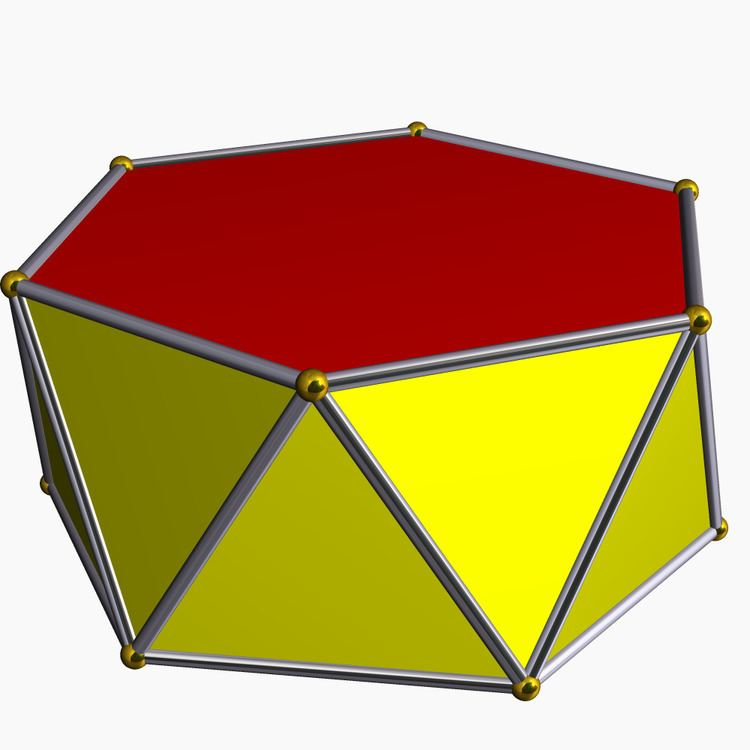# Antiprism

Updated on
Covid-19In geometry, an n-sided antiprism is a polyhedron composed of two parallel copies of some particular n-sided polygon, connected by an alternating band of triangles. Antiprisms are a subclass of the prismatoids and are a (degenerate) type of snub polyhedra.

## Contents

Antiprisms are similar to prisms except the bases are twisted relative to each other, and that the side faces are triangles, rather than quadrilaterals.

In the case of a regular n-sided base, one usually considers the case where its copy is twisted by an angle 180°/n. Extra regularity is obtained when the line connecting the base centers is perpendicular to the base planes, making it a right antiprism. As faces, it has the two n-gonal bases and, connecting those bases, 2n isosceles triangles.

## Uniform antiprism

A uniform antiprism has, apart from the base faces, 2n equilateral triangles as faces. As a class, the uniform antiprisms form an infinite series of vertex-uniform polyhedra, as do the uniform prisms. For n = 2 we have as degenerate case the regular tetrahedron as a digonal antiprism, and for n = 3 the non-degenerate regular octahedron as a triangular antiprism.

The dual polyhedra of the antiprisms are the trapezohedra. Their existence was discussed and their name was coined by Johannes Kepler, though it is possible that they were previously known to Archimedes as they satisfy the same conditions on vertices as the Archimedean solids.

## Cartesian coordinates

Cartesian coordinates for the vertices of a right antiprism with n-gonal bases and isosceles triangles are

( cos k π n , sin k π n , ( 1 ) k h )

with k ranging from 0 to 2n − 1; if the triangles are equilateral,

2 h 2 = cos π n cos 2 π n .

## Volume and surface area

Let a be the edge-length of a uniform antiprism. Then the volume is

V = n 4 cos 2 π 2 n 1 sin 3 π 2 n 12 sin 2 π n a 3

and the surface area is

A = n 2 ( cot π n + 3 ) a 2 .

## Related polyhedra

There are an infinite set of truncated antiprisms, including a lower-symmetry form of the truncated octahedron (truncated triangular antiprism). These can be alternated to create snub antiprisms, two of which are Johnson solids, and the snub triangular antiprism is a lower symmetry form of the icosahedron.

## Symmetry

The symmetry group of a right n-sided antiprism with regular base and isosceles side faces is Dnd of order 4n, except in the case of a tetrahedron, which has the larger symmetry group Td of order 24, which has three versions of D2d as subgroups, and the octahedron, which has the larger symmetry group Oh of order 48, which has four versions of D3d as subgroups.

The symmetry group contains inversion if and only if n is odd.

The rotation group is Dn of order 2n, except in the case of a tetrahedron, which has the larger rotation group T of order 12, which has three versions of D2 as subgroups, and the octahedron, which has the larger rotation group O of order 24, which has four versions of D3 as subgroups.

## Star antiprism

Uniform star antiprisms are named by their star polygon bases, {p/q}, and exist in prograde and retrograde (crossed) solutions. Crossed forms have intersecting vertex figures, and are denoted by inverted fractions, p/(p - q) instead of p/q, e.g. 5/3 instead of 5/2.

In the retrograde forms but not in the prograde forms, the triangles joining the star bases intersect the axis of rotational symmetry.

Some retrograde star antiprisms with regular star polygon bases cannot be constructed with equal edge lengths, so are not uniform polyhedra. Star antiprism compounds also can be constructed where p and q have common factors; thus a 10/4 antiprism is the compound of two 5/2 star antiprisms.

• Prism
• Apeirogonal antiprism
• Grand antiprism – a four-dimensional polytope
• One World Trade Center, a building consisting primarily of an elongated square antiprism
• ## References

Similar Topics
Black Like Me (film)
Dick Nen
Daniel Richler
Topics